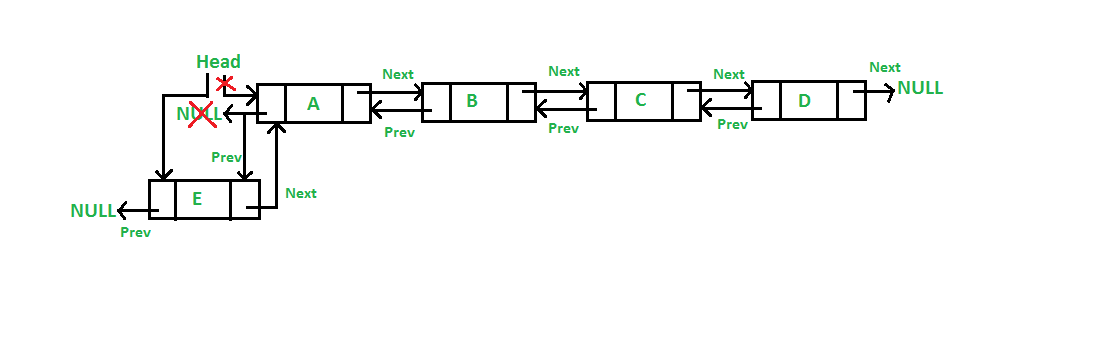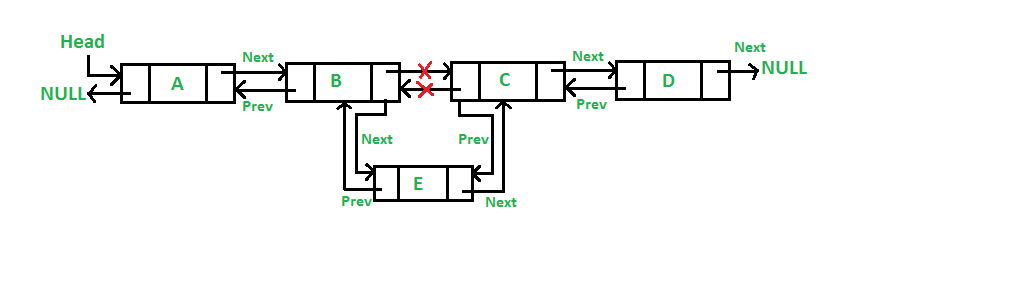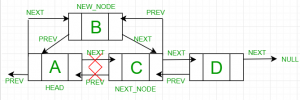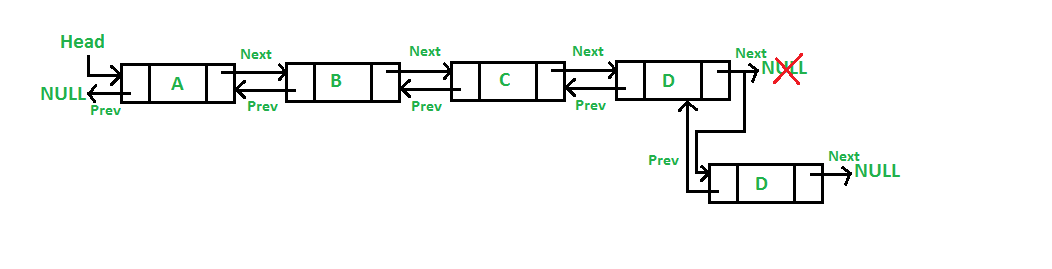Open In App
Related Articles

# Insertion in a Doubly Linked List

Inserting a new node in a doubly linked list is very similar to inserting new node in linked list. There is a little extra work required to maintain the link of the previous node. A node can be inserted in a Doubly Linked List in four ways:

• At the front of the DLL.
• In between two nodes
• After a given node.
• Before a given node.
• At the end of the DLL.

## Add a node at the front in a Doubly Linked List:

The new node is always added before the head of the given Linked List. The task can be performed by using the following 5 steps:

1. Firstly, allocate a new node (say new_node).
2. Now put the required data in the new node.
3. Make the next of new_node point to the current head of the doubly linked list.
4. Make the previous of the current head point to new_node.
5. Lastly, point head to new_node.

Illustration:

See the below illustration where E is being inserted at the beginning of the doubly linked list.Below is the implementation of the 5 steps to insert a node at the front of the linked list:

## C

 `// Given a reference (pointer to pointer) to the head``// of a list and an int, inserts a new node``// on the front of the list.``void` `push(``struct` `Node** head_ref, ``int` `new_data)``{``    ``// 1. allocate node``    ``struct` `Node* new_node``        ``= (``struct` `Node*)``malloc``(``sizeof``(``struct` `Node));` `    ``// 2. put in the data``    ``new_node->data = new_data;` `    ``// 3. Make next of new node as head and previous as NULL``    ``new_node->next = (*head_ref);``    ``new_node->prev = NULL;` `    ``// 4. change prev of head node to new node``    ``if` `((*head_ref) != NULL)``        ``(*head_ref)->prev = new_node;` `    ``// 5. move the head to point to the new node``    ``(*head_ref) = new_node;``}`

## C++

 `// Given a reference (pointer to pointer) to the head``// of a list and an int, inserts a new node``// on the front of the list.``void` `push(Node** head_ref, ``int` `new_data)``{``    ``// 1. allocate node``    ``Node* new_node = ``new` `Node();` `    ``// 2. put in the data``    ``new_node->data = new_data;` `    ``// 3. Make next of new node as head``    ``// and previous as NULL``    ``new_node->next = (*head_ref);``    ``new_node->prev = NULL;` `    ``// 4. change prev of head node to new node``    ``if` `((*head_ref) != NULL)``        ``(*head_ref)->prev = new_node;` `    ``// 5. move the head to point to the new node``    ``(*head_ref) = new_node;``}` `// This code is contributed by shivanisinghss2110`

## Java

 `// Adding a node at the front of the list``public` `void` `push(``int` `new_data)``{``    ``// 1. allocate node``    ``// 2. put in the data */``    ``Node new_Node = ``new` `Node(new_data);` `    ``// 3. Make next of new node as head and previous as NULL``    ``new_Node.next = head;``    ``new_Node.prev = ``null``;` `    ``// 4. change prev of head node to new node``    ``if` `(head != ``null``)``        ``head.prev = new_Node;` `    ``// 5. move the head to point to the new node``    ``head = new_Node;``}`

## Python3

 `# Adding a node at the front of the list``def` `push(``self``, new_data):` `    ``# 1 & 2: Allocate the Node & Put in the data``    ``new_node ``=` `Node(data``=``new_data)` `    ``# 3. Make next of new node as head and previous as NULL``    ``new_node.``next` `=` `self``.head``    ``new_node.prev ``=` `None` `    ``# 4. change prev of head node to new node``    ``if` `self``.head ``is` `not` `None``:``        ``self``.head.prev ``=` `new_node` `    ``# 5. move the head to point to the new node``    ``self``.head ``=` `new_node` `# This code is contributed by jatinreaper`

## C#

 `// Adding a node at the front of the list``public` `void` `push(``int` `new_data)``{``    ``// 1. allocate node``    ``// 2. put in the data``    ``Node new_Node = ``new` `Node(new_data);` `    ``// 3. Make next of new node as head and previous as NULL``    ``new_Node.next = head;``    ``new_Node.prev = ``null``;` `    ``// 4. change prev of head node to new node``    ``if` `(head != ``null``)``        ``head.prev = new_Node;` `    ``// 5. move the head to point to the new node``    ``head = new_Node;``}` `// This code is contributed by aashish1995`

## Javascript

 `// Adding a node at the front of the list``function` `push(new_data)``{``    ``// 1. allocate node``    ``// 2. put in the data``    ``let new_Node = ``new` `Node(new_data);` `    ``// 3. Make next of new node as head and previous as NULL``    ``new_Node.next = head;``    ``new_Node.prev = ``null``;` `    ``// 4. change prev of head node to new node``    ``if` `(head != ``null``)``        ``head.prev = new_Node;` `    ``// 5. move the head to point to the new node``    ``head = new_Node;``}` `// This code is contributed by saurabh_jaiswal.`

Time Complexity: O(1)
Auxiliary Space: O(1)

## Add a node in between two nodes:

It is further classified into the following two parts:

### Add a node after a given node in a Doubly Linked List:

We are given a pointer to a node as prev_node, and the new node is inserted after the given node. This can be done using the following 6 steps:

1. Firstly create a new node (say new_node).
2. Now insert the data in the new node.
3. Point the next of new_node to the next of prev_node.
4. Point the next of prev_node to new_node.
5. Point the previous of new_node to prev_node.
6. Change the pointer of the new node’s previous pointer to new_node.

Illustration:

See the below illustration where ‘E‘ is being inserted after ‘B‘.Below is the implementation of the 7 steps to insert a node after a given node in the linked list:

## C

 `// Given a node as prev_node, insert a new node``// after the given node``void` `insertAfter(``struct` `Node* prev_node, ``int` `new_data)``{``    ``// Check if the given prev_node is NULL``    ``if` `(prev_node == NULL) {``        ``printf``(``"the given previous node cannot be NULL"``);``        ``return``;``    ``}` `    ``// 1. allocate new node``    ``struct` `Node* new_node``        ``= (``struct` `Node*)``malloc``(``sizeof``(``struct` `Node));` `    ``// 2. put in the data``    ``new_node->data = new_data;` `    ``// 3. Make next of new node as next of prev_node``    ``new_node->next = prev_node->next;` `    ``// 4. Make the next of prev_node as new_node``    ``prev_node->next = new_node;` `    ``// 5. Make prev_node as previous of new_node``    ``new_node->prev = prev_node;` `    ``// 6. Change previous of new_node's next node``    ``if` `(new_node->next != NULL)``        ``new_node->next->prev = new_node;``}`

## C++

 `// Given a node as prev_node, insert a new node``// after the given node``void` `insertAfter(Node* prev_node, ``int` `new_data)``{``    ``// Check if the given prev_node is NULL``    ``if` `(prev_node == NULL) {``        ``cout << ``"the given previous node cannot be NULL"``;``        ``return``;``    ``}` `    ``// 1. allocate new node``    ``Node* new_node = ``new` `Node();` `    ``// 2. put in the data``    ``new_node->data = new_data;` `    ``// 3. Make next of new node as next of prev_node``    ``new_node->next = prev_node->next;` `    ``// 4. Make the next of prev_node as new_node``    ``prev_node->next = new_node;` `    ``// 5. Make prev_node as previous of new_node``    ``new_node->prev = prev_node;` `    ``// 6. Change previous of new_node's next node``    ``if` `(new_node->next != NULL)``        ``new_node->next->prev = new_node;``}` `// This code is contributed by shivanisinghss2110.`

## Java

 `// Given a node as prev_node, insert a new node``// after the given node``public` `void` `InsertAfter(Node prev_Node, ``int` `new_data)``{``    ``// Check if the given prev_node is NULL``    ``if` `(prev_Node == ``null``) {``        ``System.out.println(``            ``"The given previous node cannot be NULL "``);``        ``return``;``    ``}` `    ``// 1. allocate node``    ``// 2. put in the data``    ``Node new_node = ``new` `Node(new_data);` `    ``// 3. Make next of new node as next of prev_node``    ``new_node.next = prev_Node.next;` `    ``// 4. Make the next of prev_node as new_node``    ``prev_Node.next = new_node;` `    ``// 5. Make prev_node as previous of new_node``    ``new_node.prev = prev_Node;` `    ``// 6. Change previous of new_node's next node``    ``if` `(new_node.next != ``null``)``        ``new_node.next.prev = new_node;``}`

## Python3

 `# Given a node as prev_node, insert``# a new node after the given node`  `def` `insertAfter(``self``, prev_node, new_data):` `    ``# Check if the given prev_node is NULL``    ``if` `prev_node ``is` `None``:``        ``print``(``"This node doesn't exist in DLL"``)``        ``return` `    ``# 1. allocate node  &``    ``# 2. put in the data``    ``new_node ``=` `Node(data``=``new_data)` `    ``# 3. Make next of new node as next of prev_node``    ``new_node.``next` `=` `prev_node.``next` `    ``# 4. Make the next of prev_node as new_node``    ``prev_node.``next` `=` `new_node` `    ``# 5. Make prev_node as previous of new_node``    ``new_node.prev ``=` `prev_node` `    ``# 6. Change previous of new_node's next node``    ``if` `new_node.``next` `is` `not` `None``:``        ``new_node.``next``.prev ``=` `new_node` `#  This code is contributed by jatinreaper`

## C#

 `// Given a node as prev_node, insert a new node``// after the given node``public` `void` `InsertAfter(Node prev_Node, ``int` `new_data)``{``    ``// Check if the given prev_node is NULL``    ``if` `(prev_Node == ``null``) {``        ``Console.WriteLine(``            ``"The given previous node cannot be NULL "``);``        ``return``;``    ``}` `    ``// 1. allocate node``    ``// 2. put in the data``    ``Node new_node = ``new` `Node(new_data);` `    ``// 3. Make next of new node as next of prev_node``    ``new_node.next = prev_Node.next;` `    ``// 4. Make the next of prev_node as new_node``    ``prev_Node.next = new_node;` `    ``// 5. Make prev_node as previous of new_node``    ``new_node.prev = prev_Node;` `    ``// 6. Change previous of new_node's next node``    ``if` `(new_node.next != ``null``)``        ``new_node.next.prev = new_node;``}` `// This code is contributed by aashish1995`

## Javascript

 ``

Time Complexity: O(1)
Auxiliary Space: O(1)

### Add a node before a given node in a Doubly Linked List:

Let the pointer to this given node be next_node. This can be done using the following 6 steps.

1. Allocate memory for the new node, let it be called new_node.
2. Put the data in new_node.
3. Set the previous pointer of this new_node as the previous node of the next_node.
4. Set the previous pointer of the next_node as the new_node.
5. Set the next pointer of this new_node as the next_node.
6. Now set the previous pointer of new_node.
• If the previous node of the new_node is not NULL, then set the next pointer of this previous node as new_node.
• Else, if the prev of new_node is NULL, it will be the new head node.

Illustration:

See the below illustration where ‘B‘ is being inserted before ‘C‘.Below is the implementation of the above approach.

## C

 `// Given a node as prev_node, insert a new node``// after the given node``void` `insertBefore(``struct` `Node* next_node, ``int` `new_data)``{``    ``// Check if the given next_node is NULL``    ``if` `(next_node == NULL) {``        ``printf``(``"the given next node cannot be NULL"``);``        ``return``;``    ``}` `    ``// 1. Allocate new node``    ``struct` `Node* new_node``        ``= (``struct` `Node*)``malloc``(``sizeof``(``struct` `Node));` `    ``// 2. Put in the data``    ``new_node->data = new_data;` `    ``// 3. Make previous of new node as previous of next_node``    ``new_node->prev = next_node->prev;` `    ``// 4. Make the previous of next_node as new_node``    ``next_node->prev = new_node;` `    ``// 5. Make next_node as next of new_node``    ``new_node->next = next_node;` `    ``// 6. Change next of new_node's previous node``    ``if` `(new_node->prev != NULL)``        ``new_node->prev->next = new_node;``    ``else``        ``head = new_node;``}`

## C++

 `// Given a node as prev_node, insert a new node``// after the given node``void` `insertBefore(Node* next_node, ``int` `new_data)``{``    ``// Check if the given next_node is NULL``    ``if` `(next_node == NULL) {``        ``printf``(``"the given next node cannot be NULL"``);``        ``return``;``    ``}` `    ``// 1. Allocate new node``    ``Node* new_node = ``new` `Node();` `    ``// 2. Put in the data``    ``new_node->data = new_data;` `    ``// 3. Make previous of new node as previous of next_node``    ``new_node->prev = next_node->prev;` `    ``// 4. Make the previous of next_node as new_node``    ``next_node->prev = new_node;` `    ``// 5. Make next_node as next of new_node``    ``new_node->next = next_node;` `    ``// 6. Change next of new_node's previous node``    ``if` `(new_node->prev != NULL)``        ``new_node->prev->next = new_node;``    ``else``        ``head = new_node;``}`

## Java

 `// Given a node as prev_node, insert a new node``// after the given node``public` `void` `InsertBefore(Node next_Node, ``int` `new_data)``{``    ``// Check if the given next_node is NULL``    ``if` `(next_Node == ``null``) {``        ``System.out.println(``            ``"The given next node cannot be NULL "``);``        ``return``;``    ``}` `    ``// 1. Allocate node``    ``// 2. Put in the data``    ``Node new_node = ``new` `Node(new_data);` `    ``// 3. Make previous of new node as previous of prev_node``    ``new_node.prev = next_Node.prev;` `    ``// 4. Make the prev of next_node as new_node``    ``next_Node.prev = new_node;` `    ``// 5. Make next_node as next of new_node``    ``new_node.next = next_Node;` `    ``// 6. Change next of new_node's previous node``    ``if` `(new_node.prev != ``null``)``        ``new_node.prev.next = new_node;``    ``else``        ``head = new_node;``}`

## Python3

 `# Given a node as prev_node, insert``# a new node after the given node`  `def` `insertAfter(``self``, next_node, new_data):` `    ``# Check if the given next_node is NULL``    ``if` `next_node ``is` `None``:``        ``print``(``"This node doesn't exist in DLL"``)``        ``return` `    ``# 1. Allocate node  &``    ``# 2. Put in the data``    ``new_node ``=` `Node(data``=``new_data)` `    ``# 3. Make previous of new node as previous of prev_node``    ``new_node.prev ``=` `next_node.prev` `    ``# 4. Make the previous of next_node as new_node``    ``next_node.prev ``=` `new_node` `    ``# 5. Make next_node as next of new_node``    ``new_node.``next` `=` `next_node` `    ``# 6. Change next of new_node's previous node``    ``if` `new_node.prev ``is` `not` `None``:``        ``new_node.prev.``next` `=` `new_node``    ``else``:``        ``head ``=` `new_node` `#  This code is contributed by jatinreaper`

## C#

 `// Given a node as prev_node, insert a new node``// after the given node``public` `void` `InsertAfter(Node next_Node, ``int` `new_data)``{``    ``// Check if the given next_node is NULL``    ``if` `(next_Node == ``null``) {``        ``Console.WriteLine(``            ``"The given next node cannot be NULL "``);``        ``return``;``    ``}` `    ``// 1. Allocate node``    ``// 2. Put in the data``    ``Node new_node = ``new` `Node(new_data);` `    ``// 3. Make previous of new node as previous of next_node``    ``new_node.prev = next_Node.prev;` `    ``// 4. Make the previous of next_node as new_node``    ``next_Node.prev = new_node;` `    ``// 5. Make next_node as next of new_node``    ``new_node.next = next_Node;` `    ``// 6. Change next of new_node's previous node``    ``if` `(new_node.prev != ``null``)``        ``new_node.prev.next = new_node;``    ``else``        ``head = new_node;``}` `// This code is contributed by aashish1995`

## Javascript

 ``

Time Complexity: O(1)
Auxiliary Space: O(1)

## Add a node at the end in a Doubly Linked List:

The new node is always added after the last node of the given Linked List. This can be done using the following 7 steps:

1. Create a new node (say new_node).
2. Put the value in the new node.
3. Make the next pointer of new_node as null.
4. If the list is empty, make new_node as the head.
5. Otherwise, travel to the end of the linked list.
6. Now make the next pointer of last node point to new_node.
7. Change the previous pointer of new_node to the last node of the list.

Illustration:

See the below illustration where ‘D‘ is inserted at the end of the linked list.Below is the implementation of the 7 steps to insert a node at the end of the linked list:

## C

 `// Given a reference (pointer to pointer) to the head``// of a DLL and an int, appends a new node at the end``void` `append(``struct` `Node** head_ref, ``int` `new_data)``{``    ``// 1. allocate node``    ``struct` `Node* new_node``        ``= (``struct` `Node*)``malloc``(``sizeof``(``struct` `Node));` `    ``struct` `Node* last = *head_ref; ``/* used in step 5*/` `    ``// 2. put in the data``    ``new_node->data = new_data;` `    ``// 3. This new node is going to be the last node, so``    ``// make next of it as NULL``    ``new_node->next = NULL;` `    ``// 4. If the Linked List is empty, then make the new``    ``// node as head``    ``if` `(*head_ref == NULL) {``        ``new_node->prev = NULL;``        ``*head_ref = new_node;``        ``return``;``    ``}` `    ``// 5. Else traverse till the last node``    ``while` `(last->next != NULL)``        ``last = last->next;` `    ``// 6. Change the next of last node``    ``last->next = new_node;` `    ``// 7. Make last node as previous of new node``    ``new_node->prev = last;` `    ``return``;``}`

## C++

 `// Given a reference (pointer to pointer) to the head``// of a DLL and an int, appends a new node at the end``void` `append(Node** head_ref, ``int` `new_data)``{``    ``// 1. allocate node``    ``Node* new_node = ``new` `Node();` `    ``Node* last = *head_ref; ``/* used in step 5*/` `    ``// 2. put in the data``    ``new_node->data = new_data;` `    ``// 3. This new node is going to be the last node, so``    ``// make next of it as NULL``    ``new_node->next = NULL;` `    ``// 4. If the Linked List is empty, then make the new``    ``// node as head``    ``if` `(*head_ref == NULL) {``        ``new_node->prev = NULL;``        ``*head_ref = new_node;``        ``return``;``    ``}` `    ``// 5. Else traverse till the last node``    ``while` `(last->next != NULL)``        ``last = last->next;` `    ``// 6. Change the next of last node``    ``last->next = new_node;` `    ``// 7. Make last node as previous of new node``    ``new_node->prev = last;` `    ``return``;``}` `// This code is contributed by shivanisinghss2110`

## Java

 `// Add a node at the end of the list``void` `append(``int` `new_data)``{``    ``// 1. allocate node``    ``// 2. put in the data``    ``Node new_node = ``new` `Node(new_data);` `    ``Node last = head; ``/* used in step 5*/` `    ``// 3. This new node is going to be the last node, so``    ``// make next of it as NULL``    ``new_node.next = ``null``;` `    ``// 4. If the Linked List is empty, then make the new``    ``// node as head``    ``if` `(head == ``null``) {``        ``new_node.prev = ``null``;``        ``head = new_node;``        ``return``;``    ``}` `    ``// 5. Else traverse till the last node``    ``while` `(last.next != ``null``)``        ``last = last.next;` `    ``// 6. Change the next of last node``    ``last.next = new_node;` `    ``// 7. Make last node as previous of new node``    ``new_node.prev = last;``}`

## Python3

 `# Add a node at the end of the DLL``def` `append(``self``, new_data):` `    ``# 1. allocate node``    ``# 2. put in the data``    ``new_node ``=` `Node(data``=``new_data)``    ``last ``=` `self``.head` `    ``# 3. This new node is going to be the``    ``# last node, so make next of it as NULL``    ``new_node.``next` `=` `None` `    ``# 4. If the Linked List is empty, then``    ``#  make the new node as head``    ``if` `self``.head ``is` `None``:``        ``new_node.prev ``=` `None``        ``self``.head ``=` `new_node``        ``return` `    ``# 5. Else traverse till the last node``    ``while` `(last.``next` `is` `not` `None``):``        ``last ``=` `last.``next` `    ``# 6. Change the next of last node``    ``last.``next` `=` `new_node``    ``# 7. Make last node as previous of new node */``    ``new_node.prev ``=` `last` `#  This code is contributed by jatinreaper`

## C#

 `// Add a node at the end of the list``void` `append(``int` `new_data)``{``    ``// 1. allocate node``    ``// 2. put in the data``    ``Node new_node = ``new` `Node(new_data);` `    ``Node last = head; ``/* used in step 5*/` `    ``// 3. This new node is going``    ``//  to be the last node, so``    ``// make next of it as NULL``    ``new_node.next = ``null``;` `    ``// 4. If the Linked List is empty,``    ``// then make the new node as head``    ``if` `(head == ``null``) {``        ``new_node.prev = ``null``;``        ``head = new_node;``        ``return``;``    ``}` `    ``// 5. Else traverse till the last node``    ``while` `(last.next != ``null``)``        ``last = last.next;` `    ``// 6. Change the next of last node``    ``last.next = new_node;` `    ``// 7. Make last node as previous of new node``    ``new_node.prev = last;``}` `// This code is contributed by shivanisinghss2110`

## Javascript

 ``

Time Complexity: O(n)
Auxiliary Space: O(1)

Related Articles: